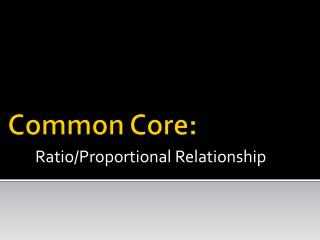Download PresentationCommon Core:

# Common Core:

Download Presentation## Common Core:

- - - - - - - - - - - - - - - - - - - - - - - - - - - E N D - - - - - - - - - - - - - - - - - - - - - - - - - - -
##### Presentation Transcript

1. Common Core: Ratio/Proportional Relationship

2. Grade 6 • 1. Understand the concept of a ratio and use ratio language to describe a ratio relationship between two quantities. • Ex: The ratio of wings beaks in the bird house at the zoo was 2:1 because for every 2 wings there was 1 beak.

3. Grade 6 • 2. Understand the concept of a unit rate a/b associated with a ratio a:b • Ex: “We paid \$75 for 15 hamburgers, which is a rate of \$5 per hamburger.”

4. Grade 6 • 3. Use ratio and rate reasoning to solve real-world and mathematical problems. • Ex: Make tables of equivalent ratio, solve unit rate problems, find a percent of a quantity as a rate per 100.

5. Problem 1 • If I can write 2 pages in 8 minutes, how many pages can I write in 12 minutes? • ¼ page per minute, so 12*(1/4) pages in 12 min. (unit rate) • 4 minutes per page, so (12/4)*1 pages in 12 min. • 2 groups of 4 minutes versus 3 groups of 4 minutes (common unit) • 8 to 12 is an increase by ½, so 2 pages goes to 3 pages

6. Grade 7 • 1. Compute unit rates associated with ratios of fractions • Including ratios of lengths, areas and other quantities measured in like or different units. • Wrote ¼ of a page in ½ an hour

7. Grade 7 • 2. Recognize and represent proportional relationships between quantities. • Decide whether two quantities are in a proportional relationship • Indentify the constant of proportionality • Explain what a point (x, y) on the graph of a proportional relationship means

8. Grade 7 • 3. Use proportional relationships to solve multistep ratio and percent problems. • Ex: Simple interest, tax, markups and markdowns, gratuities and commissions, fees, percent increase and decrease, percent error.

9. Problem 2 • Suppose I want \$10,000 at the end of the year. If I can get 10% interest, how much should I invest?